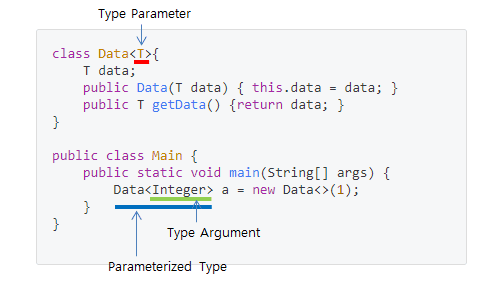Gidhub BE Developer

# Java Generics 기초 학습하기 : The Basics of Java Generics

2020-10-01
goodGid

## Java Generics

### GlossaryType Parameter

• Generic 타입을 명시하기 위한 Placeholder이다.

즉 위 코드에서 Data 클래스의 T가 이에 해당된다.

Type Argument

• 실제 Generic 타입에 명시된 타입을 의미한다.

위 코드에서는 Integer가 해당된다.

Parameterized Type

• Type argument에 의하여 Type Parameter가 치환된 전체 데이터 타입을 의미한다.

위 코드에서는 Data<Integer>가 해당된다.

### Type Erasure

``````Generics were added to Java to ensure type safety and to ensure that generics wouldn't cause overhead at runtime, the compiler applies a process called type erasure on generics at compile time.

Type erasure removes all type parameters and replaces it with their bounds or with Object if the type parameter is unbounded.

Thus the bytecode after compilation contains only normal classes, interfaces and methods thus ensuring that no new types are produced.
``````
• This is an example of type erasure:
``````public <T> List<T> genericMethod(List<T> list) {
return list.stream().collect(Collectors.toList());
}
``````
• With type erasure, the unbounded type T is replaced with Object as follows:
``````// for illustration
public List<Object> withErasure(List<Object> list) {
return list.stream().collect(Collectors.toList());
}

// which in practice results in
public List withErasure(List list) {
return list.stream().collect(Collectors.toList());
}
``````
• If the type is bounded, then the type will be replaced by the bound at compile time:
``````public <T extends Building> void genericMethod(T t) {
...
}
``````
• would change after compilation:
``````public void genericMethod(Building t) {
...
}
``````

Summary

• The situation where erasure occurs is as follows.
``````if (type parameter is unbounded) {
replace type parameter with Object
} else {
replace type parameter with their bounds
}
``````

### Generics and Primitive Data Types

• A restriction of generics in Java is that the type parameter cannot be a primitive type.

• For example, the following doesn’t compile:

``````List<int> list = new ArrayList<>();
``````
• To understand why primitive data types don’t work

let’s remember that generics are a compile-time feature

meaning the type parameter is erased and all generic types are implemented as type Object.

• As an example, let’s look at the add method of a list:

``````List<Integer> list = new ArrayList<>();
``````
• The signature of the add method is:
``````boolean add(E e);
``````
• And will be compiled to:
``````boolean add(Object e);
``````
• Therefore, type parameters must be convertible to Object.

Since primitive types don’t extend Object, we can’t use them as type parameters.

• However, Java provides boxed types for primitives, along with autoboxing and unboxing to unwrap them:

``````Integer a = 17;
int b = a;
``````
• So, if we want to create a list which can hold integers, we can use the wrapper:
``````List<Integer> list = new ArrayList<>();
int first = list.get(0);
``````
• The compiled code will be the equivalent of:
``````List list = new ArrayList<>();
int first = ((Integer) list.get(0)).intValue();
``````
• Future versions of Java might allow primitive data types for generics.

Project Valhalla aims at improving the way generics are handled.

The idea is to implement generics specialization as described in JEP 218.